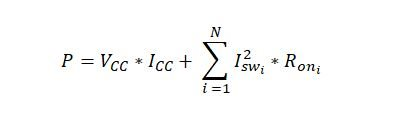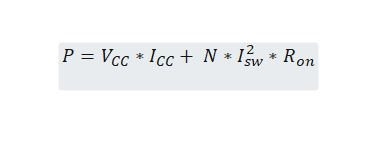• Resolved

# SN74CBTLV16212: Computing Power consumption

Part Number: SN74CBTLV16212

I want to estimate the power consumption of my design. How can I do it for sn74cbtlv16212? At page 5, ICC is stated as 10uA, but ΔICC is a bit confusing.

Plz clarify the current consumption per I/O, for 12 bit and for 24 bit applications.

Thanks

• Hi Bilal,

The delta ICC value is there to show that when the control inputs aren't VCC or GND that there will be more current drawn. The devices average power draw is 10uA that changes though depending on the signal level  at the select pins.

What is the voltage supply you are using and what is the VOH/VOL of the control signals controlling the device? With this information I will give you more of a ball part estimate.

Best,

Parker Dodson

• In reply to Parker Dodson:

Hi Dodson,

Supply voltage is 3.3V. Control Signal are coming via SN74LVC1G04 (operating @ 3.3V) , generated at PCF8575C  (operating @ 3.3V) .

Regards,

Bilal

Hi Bilal,

With a Low current draw to the control pins the signal level on the pin can be assumed to be ~VCC or GND. So the power supply current can be approximated as the 10uA value because this is testing supply current with all control pins at ~VCC or GND to ensure the lowest current draw.

Based on that the power consumption due to supply current you are looking at ~33uA + power losses due to resistance of switch (shown how to calculate below)

However for every control signal that dips below VCC there will be more supply current - absolute worst case I'd see for your setup is most like 300uA (max) for every control pin that is not ~VCC or ~GND so this would be ~33uA + 300uA(max) * M + Losses from Switch - where M is the number of  select pins that are not at ~VCC or GND which I don't see to be to be a problem in the application - unless the inverters are also feeding current to more places than the control pins because more current drawn from the inverter could cause the select voltage to dip and cause an increase of supply current.

The other piece of power consumption is going to be due resistive losses of the mux itself.

The power consumption formula is as follows:Where N is the number of inputs you have (12 or 24) and I_sw is the current being ran through the switch, which depends on the load,  and the on resistance of the switch that is being used which will vary with input voltage. The formula can be simplified as the following if you assume the average Ron and switch current to be approximately equal for every channel that is activated.Where N is the number of channels being used (12 or 24).

If you have other questions or if something wasn't clear please let me know!

Best,

Parker Dodson

• In reply to Parker Dodson:

Hi Dodson,

Its clear. Thanks a lot!

Best Regards,

Bilal